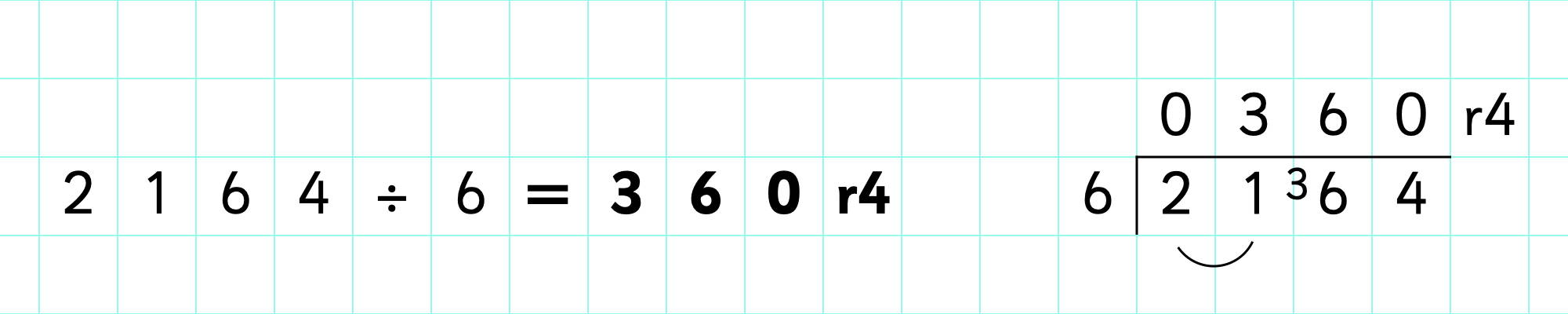# Bus Stop Method Division

## What is bus stop division?

The bus stop method is a formal method of division, so called because the layout of the calculation looks like a bus stop. It is also known as short division.

With the bus stop method, the dividend (the number being divided) is under the shelter of the bus stop, while the divisor (the number the dividend is divided by) is outside the bus stop. The quotient (the answer to the calculation) is then recorded above the bus stop.## How to do bus stop division:

The first step is to set out your calculation correctly. It can help to use squared paper to make sure that your place value columns don't get mixed up. For example, if you have the calculation 75 ÷ 3 you would set out your calculation like this: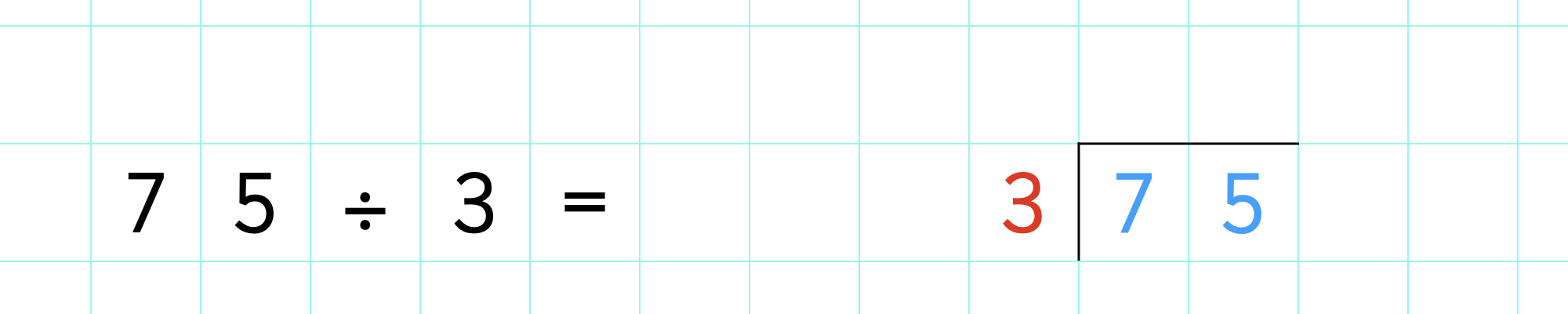We then divide the dividend by the divisor one digit at a time, always starting with the digit closest to the bus stop. This is always the number with the highest place value, In this case, we start by dividing '7' by '3'. There are 2 lots of 3 in 7, so we record this above the seven, like this: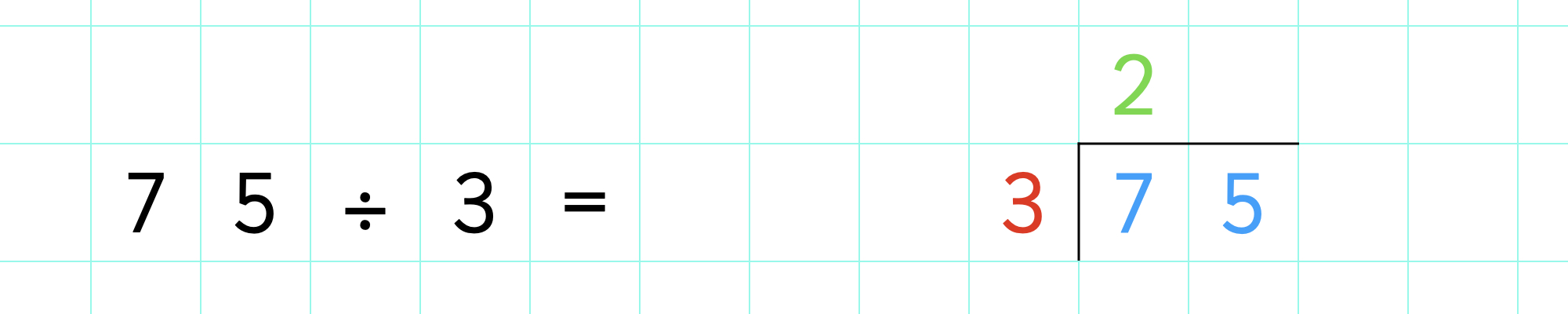However, seven doesn't divide into three exactly; there is one 'left over'. This is known as a remainder. In this case, the remainder shows that there is one ten left over after dividing 7 tens by 3. We record this remainder next to the following digit of the dividend: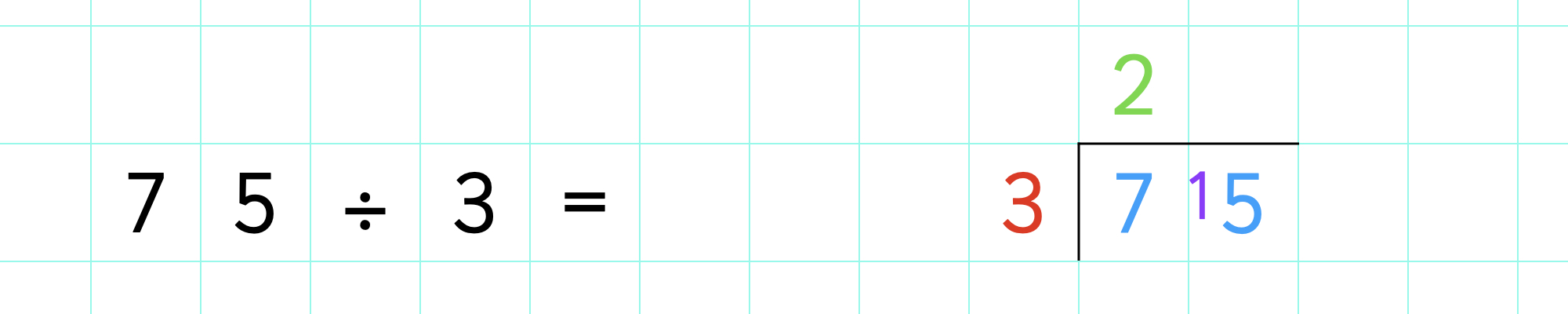The next step is to divide the next digit of the dividend by the divisor. Because we now have a remainder (the one ten we had left over), we have to divide '15' by '3', instead of '5'. 15 divided by 3 is 5, so we record this above the ones number in the dividend: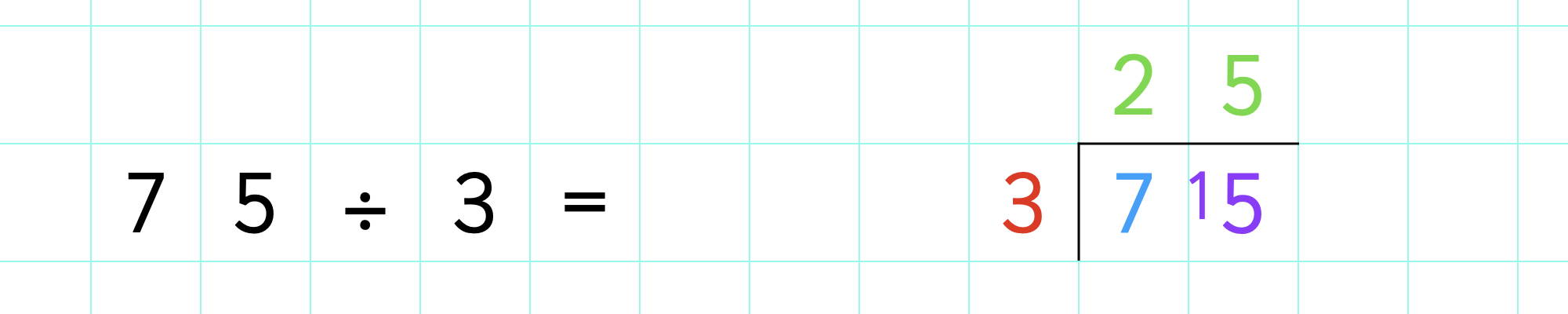There is nothing else to divide so we know we now have our answer of 23: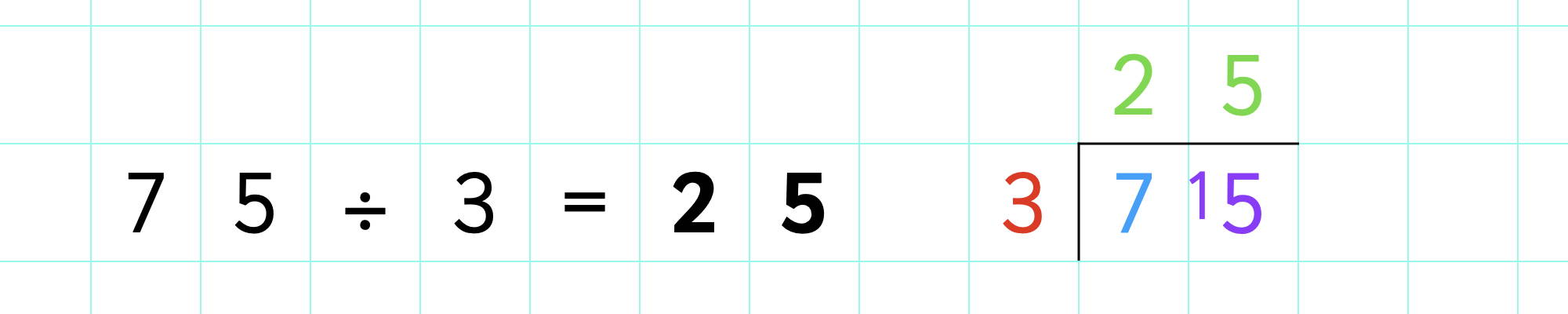And that's how to solve bus method division problems! You can use the bus stop method to solve any calculation with a one-digit divisor, with a dividend of any size.

Let's look at another example...

We start by laying out our calculation correctly: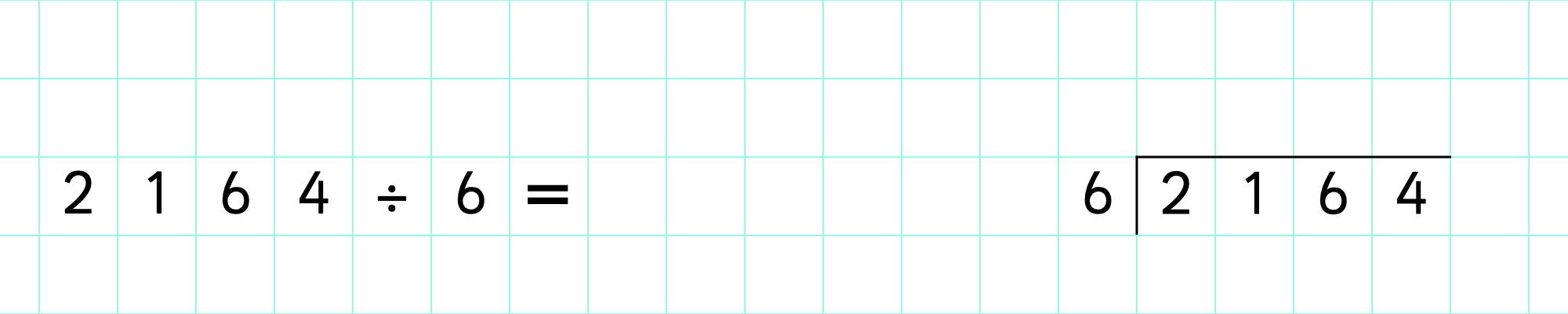Next, you need to divide 2 by 6 (the digit of the dividend nearest the bus stop). However, there are no sixes in two, so we need to include the next digit too so that we have a divisable number:

We'll divide 21 by 6 instead: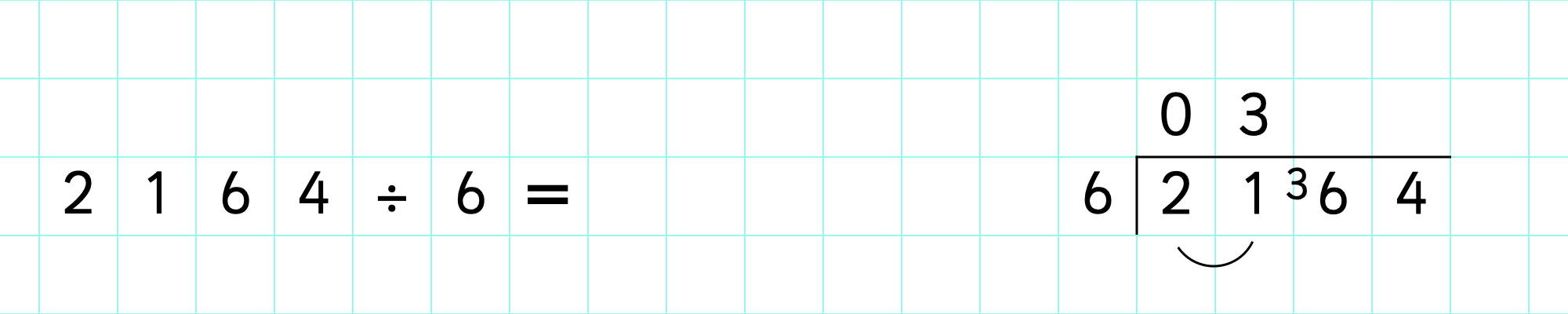21 divided by 6 is 3 with 3 left over, so we will record the remainder next to the following digit of the dividend.

Now, we need to divide 36 by 6. 36 divided by 6 is 6 exactly: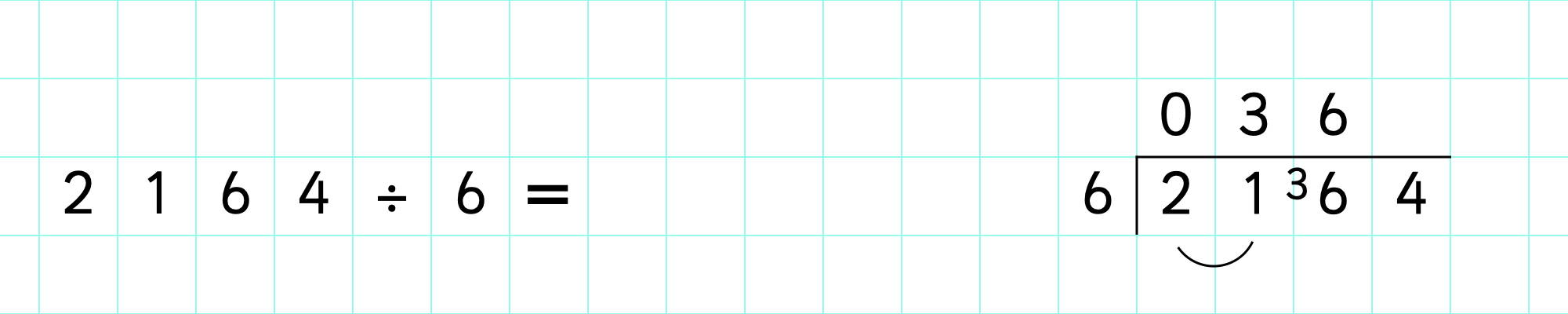Finally, we need to divide 4 by 6. There are no sixes in four, so we record '0' in the ones column.

However, we still need to account for that four, so we add it as a remainder to the calculation: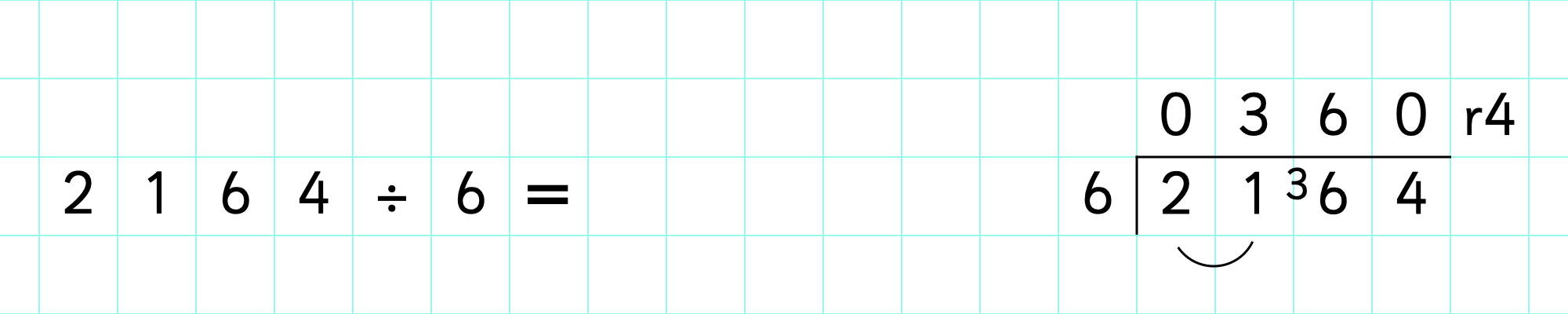We now have the answer to the calculation: